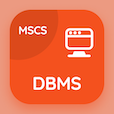Cambridge Online Courses (GCE)

A Level Physics Quizzes

A Level Physics Quiz PDF - Complete

# Orbiting Charges Multiple Choice Questions p. 113

Study Orbiting Charges multiple choice questions and answers, orbiting charges quiz answers PDF 113 to study A Level Physics course online. Charged Particles MCQ trivia questions, orbiting charges Multiple Choice Questions (MCQ) for online college degrees. "Orbiting Charges MCQ" PDF eBook: x-ray images, compression and tensile force, magnetic field in physics, acceleration calculations, orbiting charges test prep for colleges offering online degree programs.

"According to the equation ‘r = (mv)⁄(Be)’, the faster moving particles" MCQ PDF: move straight, move in smaller circle, move in bigger circle, and move randomly for best online ACT prep class. Learn charged particles questions and answers to improve problem solving skills for 2 year online degrees.

## Orbiting Charges Questions and Answers MCQs

MCQ: According to the equation ‘r = (mv)⁄(Be)’, the faster moving particles

move in smaller circle
move straight
move in bigger circle
move randomly

MCQ: If a car starting from rest reaches a velocity of 18 ms-1 after 6.0 s then its acceleration is

1 ms-2
2 ms-2
3 ms-2
4 ms-2

MCQ: A flat coil and solenoid has

different fields
same physical properties
same fields
same chemical properties

MCQ: Extension and applied force are

directly proportional
inversely proportional
are independent of each other
inversely related

MCQ: Type of x-rays used to detect break in bone is

hard
soft
both A and B
moderate

### More Quizzes from A Level Physics Course